# Table Of Contents :

• Introduction.
• Business Problem .
• ML Formulation and Constraints.
• Data Overview
• Performance Metrics.
• Data Preprocessing.
• Exploratory Data Analysis
• Feature Engineering
• Different Encoding strategies.
• Training Different Models.
• Model Comparison.
• Prediction Intervals.
• Model Deployment
• Future Work.
• Profiles
• References.

# 1. Introduction :

Services Now is a software company whose core business was to deal with management of incident, problem and change IT operational events, the current data set consists of incidents recorded in an IT company through ServiceNow platform.

# 2.Business Problem And Constraint

Ticketing system is like a bridge between customers and the service company to deal with the issues faced by customers. Whenever an issue is observed, the customer reports it and an incident regarding that is recorded in the ticketing system. Ticketing system helps to assign the ticket to a particular group based on the label and priority, it also comes in handy when there are huge tickets raised by customers regarding a specific issue, it segments the issue and assigns it to a particular group.

All is well and good but we do not know how much time it will take to resolve the ticket, the customer waits until the ticket is resolved, sometimes it might be longer than expected which can even make them lose the trust on the service company, if we can predict the time of closing the ticket, we can tell the time of closing to the customers beforehand which will be useful to the customers and the company too.

# 3. ML Formulation and Constraints:

We have closed_at time and opened_at time as the features, if we take the difference of both the features, we will get time taken to close a ticket in seconds, as they are continuous values, it can be posed as a regression problem. In the data set we have to predict resolved_at and closed_at features, so this can be treated as a multi output regression problem.

. No latency requirement

. Minimize the loss between actual and predicted values

# 4. Data Overview

The data set is taken from Kaggle, we have given two target variables and 34 independent variables. Link to data set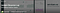Incident Response log Kaggle dataset
• There are 4 Booleans, 3 integers, 3 date-time, 1 identifier, 23 categorical and 2 dependent features, there are 119998 data points.
• Each incident is recorded with a unique ID, each id is having multiple logs or rows of data, describing each log with multiple features
• Data is anonymized for privacy, i.e. exact information is not there in the data, original data is either hide or removed from the logs
• Some of the features are having ? symbol, indicating data is unknown or missing, 4 of the features are having 99% missing data, as we cannot get any information from them, we can remove them. There are no missing values in numeric features.
• Features like caller_id, opened_by, sys_updated_by are having ids respective to the user or the caller. We cannot take them as numeric data, we need to take each ID as a separate category.
• The dependent features closed_at and resolved_at are highly correlated.

# 5. Performance Metrics

Here we have a regression problem and we need to minimize the loss between actual and predicted time, so we can take metrics like MAE, MSE, RMSE etc. here we would like to penalize the errors more so we can take MSE as the metric

It calculates the square of the difference between actual and predicted values and divides them with total data points. If there is a difference between actual and predicted values, it becomes more larger value as it is squaring the difference, so it penalizes more on the erroneous points.

# 6. Data Preprocessing

The first and foremost step is to check for null values and filling them as needed. I have used missingno module to represent the missing values in the dataset.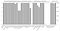Representation of missing values in bar plot

Here we can clearly see that features problem_id, rfc, vendor, caused_by, cmdb_ci are not even having 2–3 % of data points, we cannot fill them in any manner, so removing those features is the only option in our hand.

The other features which are missing are all features which are having values as ids, which need to be considered as categorical features, as these features are id’s they have high cardinality.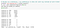Id’s of a particular feature

(please refer my git hub for detailed analysis), so currently we can take missing value as other category .

We are also having missing values in our target variable resolved_at , lets plot between target variables and see if we can get any insights.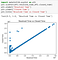Scatter plot between target variables

As you can observe from the above plot both the target features are highly positively correlated. That means as one feature increase other feature also increase. Which means the difference between them also increases , so we can take median of the difference to fill the null values.

# 7. Exploratory Data Analysis

Now lets do the univariate analysis by creating a function to plot the features against our target variables.

We have seen that our target variables are highly correlated, so I have created a function to print plot against resolved time only if it is given as true.

Above are some of the features plotted against closed time, as we discussed above there are some features with high cardinality, for those features we need to plot them differently because they will make a clutter with such amount of subcategories. So for that we can take top 5 and bottom 5 subcategories which are taking more time to close a ticket.

These are some of the features with high cardinality, I have plotted them by taking only top 5 and bottom 5 sub categories w.r.t the time they have taken to close a ticket. (please refer this for analysis of all the features).

From these plots I figured out the below key points:

• There are top 10–15 percentile categories which take more time to resolve a ticket, rest are taking a very small amount of time comparatively.
• Tickets reported by least 5 time taken caller ids are resolved, closed within a MINUTE

Now with help of box plots, let’s see how the distribution of target variables are: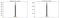Box plots of target variables

We can see that there are outliers in the data. There are chances that there are particular cases where it took more time to close or resolve, we can say them as rare points rather than outliers. so we cannot remove these points.

Now let’s create a heatmap for continuous variables and check for the correlation between them.

From this we can say that the feature sys_mod_count is most correlated, it is like 60% correlated with target variables. But we have many categorical features in the dataset, we can find the correlation between them by using a module called phik. Now lets create a heatmap for categorical features by using this phik module.

• We can see many features are correlated, active and incident state are correlated, we can take incident state as it is more correlated with target variables.
• caller_id is completely correlated with opened_by, sys_created_by, sys_updated_by, location, category, subcategory, u_symptom, assignment group, assigned_to, resolved_by features, we can keep caller_id and drop rest of the features as it is more correlated with target variables.
• contact_type and notify are correlated to each other and they are completely not correlated to target variables, better to remove these features
• impact and urgency are completely correlated, we can remove urgency feature.

# 8. Feature Engineering

We have multiple datetime features, from these we can get hour and day of the week features as new features. Now lets create hours feature from opened_at and plot them against target variable closed time

From this we can say that tickets which are opened at peak hours are taking more time to close the ticket than which are opened at non working hours (not between 8–5).

Now let’s create weak day feature from opened_at feature and plot it against closed time.

Tickets which are opened at weekdays are taking more time to close. Which are opened on weekends i.e. Saturday and Sunday are taking lesser time than prior.

Similarly we can create the day and hour features from sys_updated_at feature and create the similar plots which can be found here.

We know that week day and hour are cyclic in nature i.e. for hour feature we have a range of values 0–23, the opened ticket at 2/3/2019 23:12 is closer to 2/4/2019 0:15, if we consider these hours as is the algorithms may misinterpret them because in the usual way the difference between 0 and 23 is the most. For this we can explore trigonometrical features like sine and cosine.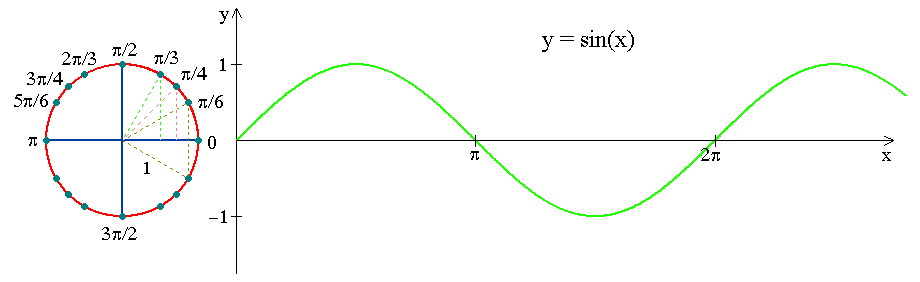Sine function (source)

We can see that the values of sine are cyclic in the range of 2pi intervals. So lets apply the same thing to our features such that we can achieve the cyclic distribution so that 0,23 will be closer. We can do the same thing to the week day feature to make them cyclic.

We have two datetime features in our dataset opened_at and sys_updated_at, now we can take the difference between them and make it as a new feature.

df2[‘updation_time’]=(df2.sys_updated_at-df2.opened_at).apply(lambda x: x.total_seconds())

Now let’s check the correlation between this feature and target variables.

We can see there is roughly 48% correlation between them which is quite good.

Now let’s see our target variables distribution.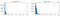Histogram of target variables

Looks like two of these are right skewed, generally applying log to these kind of distributions make them gaussian, not only this but we have time in seconds as our target variables which is a large number actually, we want to scale them so that our algorithms work better, log can also work as a scaling factor. Now let’s apply log and see the distribution.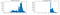Histogram of log transformed target variables

We can also try using box cox transformations to these. Which one to choose between them ? we can have Q-Q plot as an evaluator by which we can take the transformation which is closer to the gaussian distribution.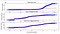Q-Q plot of target variable in different transformations

Both the transformations are almost look alike, as log is simpler than box cox, let’s use this for our modelling. Important note is we need to convert them back to their original state by applying exponential term to that.

# 9. Different Encoding strategies

Generally most of the models take only numeric features to perform their specific algorithms, so firstly we need to encode our categorical features to numerical values, for this task we can follow different approaches.

9.1 Encoding with top categories:

This is the encoding method which is used in KDD Cup Orange Challenge winners solution. Here we use the regular one hot encoding by only considering the top categorical features.

In this method we took number of data points each sub category is contributing and sorting them and taking only the top sub categories. Now in the data frame itself we will create these subcategories as new features after that we will replace them with one if the data point has that subcategory or 0 else wise.

9.2 Binary encoding :

Say we have 1000 sub categories, in regular One hot encoding it creates an additional 1000 features, which is really huge and may even lead to curse of dimensionality .

To overcome the above approach we have a technique called binary encoding, which is widely used technique for high cardinal features. The categories are first converted to numeric order starting from 1 (order is created as categories appear in a dataset and do not mean any ordinal nature). Then those integers are converted into binary code, so for example 3 becomes 011, 4 becomes 100. Then the digits of the binary number form separate columns.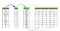Binary Encoding (source)

We can use category_encoders module to achieve this kind of encoding.

Here we apply the binary encoder from category_encoders on train data and we fit and transform that on train data and only transform on test data

9.3 Label encoding :

In this encoding, each category is assigned a value from 1 through N (here N is the number of categories for the feature. These kind of techniques are used with ordinal features where there is a relationship between the categories. We have features like impact, priority whose categories are low, medium, high. These are having a relationship like high>medium>low, so we can use this encoding for these categorical features.

Label encoder is imported from sklearn, we can fit the data on training data and use this to transform training and testing data.

# 10. Training Different Models :

Before training any model, we have our performance metric as MSE which does not have a range like R-square metric, so first let us create a bench mark MSE by creating a random model i.e. we can take the mean of target variables as predicted values and calculate MSE.

`Mean Train error: 126156.029 23908.546 Mean Test error:  126611.314 23906.956`

For calculating MSE first we need to convert the target values back to their original values by applying exponential term and add 1 to that (expm1) for predicted and transformed target variables. We got around 126k minutes of train and test MSE, now we try to build a model which gives MSE lesser than this.

As discussed above we can use the above encoding techniques. Before that we can apply CAT Boost regressor which can deal with the categorical features as is.

We have created a Cat boost regressor model and used grid search cv for hyper parameter tuning. Now calculate MSE by converting the transformed values to actual values and applying MSE on those values.

This method will predict the values with best_estimator of the model passed and then will transform these predictions back to their original values by applying exponential term on those values and then calculate MSE scores

mse_scores(grid)

`Mean Train error:  3572.996   3578.531Mean Test error:   3625.170   3565.244`

Now apply one of the encoding technique discussed above, first we will go with encoding of top categorical features and apply basic machine learning algorithms .

reg=joblib.load(‘linear.pkl’)
mse_scores(reg)

`Mean Train error:   93611.414  5804.664Mean Test error:    99611.324  6301.543`

grid_dt=joblib.load(‘decision_tree.pkl’)
mse_scores(grid_dt)

`Mean Train error:  3049.906   3013.571Mean Test error:   3270.910   3302.372`

grid_rf=joblib.load(‘random_forest.pkl’)
mse_scores(grid_rf)

`Mean Train error:  2007.350   1785.292Mean Test error:   2921.987   2896.865`

In Random Forest we have an attribute feature_importances through which we can get how important features are in predicting the target variable, we will now use this attribute and plot the feature importance's.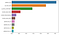Feature importance’s from Random Forest

Wow!!!! updation_time is the most important feature which we have created in our feature engineering.

Our problem is multi output regression prediction, all the above algorithms support this by default but for algorithms like XG boost we need to apply regressor chain algorithm on top of the base algorithm.

grid_xgb=joblib.load(‘xgb.pkl’)
mse_scores(grid_xgb)

`Mean Train error:  3059.083   3151.124Mean Test error:   9884.571   3294.099`

We are done with the basic models, now we will try to build custom CV regressor model. Here we will use the train data and split that into two parts D1 and D2.

Now in the D1 set use sampling with replacement and create k samples, we can consider this k as a hyper parameter.

Now we need to train these samples on different models and we need to hyper parameter these models individually.

Here we have trained a different model based on the iteration and hyper tuned it, used the D2 data for prediction on the prior best model, using these predictions as data points for a meta regressor which is also hyper tuned for every iteration , took the predictions from this and calculated the MSE scores and stored them in an array. Now lets plot both the target variables MSE scores.

That’s a serious damage !! these MSE scores are greater than random model which means it is performing worse than a random model. May be our data is unable to perform on more complex models or any other reason, we cannot pick that out but for now let’s try our luck with sk-learn’s CV regressor. This is similar to our custom built regressor model.

Here we have considered base models as ridge, Decision tree, Random Forest and KNN with ridge as a meta regressor, let’s calculate MSE for this model.

grid_ds=joblib.load(‘gird.pkl’)
mse_scores(grid_ds)

`Mean Train error:  2637.669   2517.925Mean Test error:   3591.510   3374.355`

Not bad, this looks better than catboost regressor. How ever we can still tweak with our models, we can use bagging of different base models and use mean or median as the aggregate function and calculate MSE on the final result.

`Mean Test error:  3522.687   3076.516`

We have used 4 models catboost, decision tree, random forest and ridge models which are bas models which performed better than others, now we made predictions based on this and transformed them back to their original values and took the mean of all the 4 models and calculated MSE on that.

I have implemented the other encoding strategies too but the results are not that good which can be found here

# 11. Model Comparison

Random Forest is having the least MSE score around 2.9k, so we can use this model in our deployment.

# 12. Prediction Intervals

A range of estimate is always better than a point estimate when it comes to regression problem. Prediction interval is different from confidence intervals, In confidence interval we calculate mean (generally) for the fixed data points, wherein prediction intervals we calculate values for future values of x and that too for a single data point.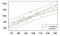Prediction Interval vs Confidence Interval

Prediction intervals are always wider than confidence intervals because the prediction interval accounts for variance in each point whereas the confidence interval considers only mean points.

Similar to the confidence interval prediction interval also has upper limit and a lower limit, for a given data point we can say that our target will lie between upper and lower limits with certain(95% generally) likelihood, but we cannot guarantee that as it completely depends on the model and data, like if the model is not predicting well or if the future data is not following the same trend.

Now if we make assumption that our x, y and our residuals follow gaussian distribution then if we want to calculate 95% prediction interval we need to calculate the standard deviation of the residuals.

`Resolved and closed prediction intervals in seconds: 49188.693 48970.000`

Here z is 1.96; z determines how many standard deviations away a data point is. To get 95% area under a normal distribution we need to have 1.96 standard deviations. We will subtract this interval from actual prediction for lower limit and add the interval to get upper limit.

# 13. Model Deployment

We will use EC2 instance in amazon with help of Flask to deploy the model. We need to create 3 files for that. First file will be used to get the inputs, we can use a normal html page to render that.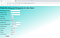Index page

Now we need to create another file namely app.py which is used to read the inputs from the index.html page and do the data preprocessing and pass the clean data to the model to predict. Here we can use random forest as our model as this gave us the least MSE score. I have used declarations in flask to navigate to the html pages and building app.py file, have a look.

Now we need create our final file which is used to give the predictions given by app.py, here also we can use html file to do that work for us.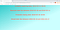Predicted closed and resolved dates

Finally we are done with the predictions. The other task is to deploy this in AWS instance, first we need to create an instance on amazon AWS, we can choose free tier ubuntu server which is free of cost which comes with 1GB of RAM. After creating the instance we need to connect to the machine with the command

ssh -i "case_1.pem" ubuntu@ec2-3-134-88-139.us-east-2.compute.amazonaws.com

At the creation of instance you will get a .pem file which is used for authentication, i had case_1.pem key and after that was my machine name.

Now after connecting you need to copy the folder into the machine, this can be done with below command

scp -r -i “case_1.pem” deployment ubuntu@ec2–3–134–88–139.us-east-2.compute.amazonaws.com:~/

I had a folder named deployment this has been moved to the machine. Now just go to the directory and execute app.py, but the connection will be lost if we close the command prompt to avoid this we can use

nohup python3 app.py &

This command will make sure to run our app.py even if we terminate our local command prompt.

App is just a click away : http://ec2-3-134-88-139.us-east-2.compute.amazonaws.com:7898/

# 14. Future Work

1. We have considered missing values in the categorical features as a separate category but there are other imputation methods like mode or KNN based imputations which may give some edge on the performance.
2. There are many more encoding techniques like frequency encoding, mean encoding etc.
3. There are multiple date time features in the data so we may try using ARIMA or deep learning models like LSTM.
4. While calculating the prediction intervals, we have assumed that data is following normal distribution, which is not true. Quantile based regressors can be used to calculate more accurate prediction intervals for any kind of distributions.

# 15. References

You can find my full code here and feel free to connect me on LinkedIn.